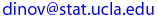# Due Date: Friday, May 23, 2003, turn in after lecture

Correct solutions to any five problems carry full credit. See the HW submission rules. On the front page include the following header.

• (HW_4_1)  [Sec. 3.5,  #69]  Suppose that p = P(male birth) = 0.5. A couple wishes to have exactly two female children in their family. The will have children until this condition is fulfilled.
(a) What is the probability that the family has x male children?
(b) What is the probability that the family has four children?
(c) What is the probability that the family has at most four children?
(d) How many male children would you expect this family to have? How many children would you expect this family to have?

• (HW_4_2)  [Sec. 3.6,  #76]  Consider writing onto a computer disk and then sending it through a certifier that counts the number of missing pulses. Suppose this number X has a Poisson distribution with parameter λ = 0.2. (Suggested in “Average
Sample Number of Semi-Curtailed Sampling Using the Poisson Distribution,” J. Quality Technology, 1983, pp. 126-129.
(a) What is the probability that a disk has exactly one missing pulse?
(b) What is the probability that a disk has at least two missing pulses?
(c) If two disks are independently selected, what is the probability that neither contains a missing pulse?

• (HW_4_3) [Sec. 4.1,  #2]  Suppose the reaction temperature (in Co) in a certain chemical process has a uniform distribution with A = −5 and B = 5.
(a) Express the probability density and the cumulative distribution function in closed forms.
(b) Compute P(X < 0).
(c) Compute P(−2.5 < X < 2.5).
(d) Compute P(−2 ≤ X ≤ 3).
(e) For k satisfying −5 < k < k + 4 < 5, compute P(k < X < k + 4).

• (HW_4_4) [Sec. 4.1,  #10 & 24] A family of pdf’s that has been used to approximate the distribution of income, city population size, and size of firms is the Pareto family. The family has two parameters, k and ϑ, both strictly positive, and the pdf is
 | kϑk / xk+1 x≥ϑ f(x; k, ϑ) = | | 0 x<ϑ
(a) Sketch the graph of f(x; k, ϑ).
(b) Verify that the total area under the for all equals 1.
(c) If the R.V. X has pdf f(x; k, ϑ) for any fixed b > ϑ obtain an expression for F(b) = P(X ≤ b).
(d) For ϑ < a < b, obtain an expression for the probability P(a ≤ X ≤ b) = F(b) − F(a).
(e) If k > 1, compute E(X).
(f) What can you say about E(X) if k = 1?
(g) If k > 2, show that σ2 = V(X) ≡ kϑ2(k − 1)−2(k − 2)−1.
(h) If k = 2, what can you say about σ2 = V(X)?

• (HW_4_5) [Sec. 4.3,  #33] Suppose the diameter at breast height (in.) of trees of a certain type is Normally distributed with µ = 8.8 and σ = 2.8, as suggested in the article “Simulating a Harvester-Forwarded Softwood Thinning” (Forest Products J., May 1997: 36-41).
(a) What is the probability that the diameter of a randomly selected tree will be at least 10 in.? Will exceed 10 in.?
(b) What is the probability that the diameter of a randomly selected tree will exceed 20 in.?
(c) What is the probability that the diameter of a randomly selected tree will be between 5 and 10 in.?
(d) What value c is such that the interval (8.8−c, 8.8+c) includes 98% of all diameter values?

• (HW_4_6) [Sec. 4.3,  #42] The Rockwell hardness of a metal is determined by impressing a hardened point into the surface of the metal and then measuring the depth of penetration of the point. Suppose the Rockwell hardness of a particular alloy is Normally distributed with mean 70 and standard deviation 3 (and that Rockwell hardness is measured on a continuous scale).

(a) If a specimen is acceptable only if its hardness is between 67 and 75, what is the probability that a randomly chosen specimen has an acceptable hardness?
(b) If the acceptable range of hardness is (70−c, 70+c), for what value of c would 95% of all specimens have acceptable hardness?
(c) If the acceptable range is as in part (a) and the hardness of each of ten randomly selected specimens is independently determined, what is the expected number of acceptable specimens among the ten?
(d) What is the probability that at most eight of the ten independently selected specimens have a hardness of less than 73.84? (Hint: Y = the number among the ten specimens with hardness less than 73.84 is a binomial variable; What is p?)

Last modified on by.

Ivo D. Dinov, Ph.D., Departments of Statistics and Neurology, UCLA School of Medicine